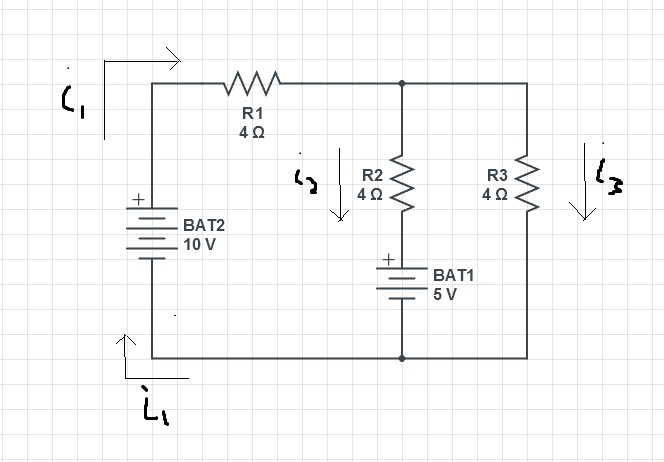# Kirchhoff's Rules. Solving for currents in circuit with two batteries

Painguy

## Homework StatementWhat are the sizes and directions of the currents through
resistors (a) R2 and (b) R3 in Fig. 27-42, where each of the three resistances is 4.0 Ω?

ΔV=IR

## The Attempt at a Solution

Equations:
1) ΔVB2 -(i1)R1 -(i2)R2 -ΔVB1=0
5V - 4(i1) - 4(i2) =0
4(i2)=5V -4(i1)

2) ΔVB2 -(i1)R1 -(i3)(R3)=0
10V -4(i1) -4(i3) =0
-4(i3)=-10V + 4(i1)

3) ΔVB1 -(i2)R2 -(i3)(R3)=0
5V + 4(i2) -4(i3) =0

4) i1=i2 + i3

5V +5V -4(i1) -10V +4(i1)=0
0=0

I'm not really sure what to do here.

Last edited:

Homework Helper

## Homework StatementWhat are the sizes and directions of the currents through
resistors (a) R2 and (b) R3 in Fig. 27-42, where each of the three resistances is 4.0 Ω?

ΔV=IR

## The Attempt at a Solution

Equations:
1) ΔVB2 -(i1)R1 -(i2)R2 -ΔVB1=0
5V - 4(i1) - 4(i2) =0
4(i2)=5V -4(i1)

2) ΔVB2 -(i1)R1 -(i3)(R3)=0
10V -4(i1) -4(i3) =0
-4(i3)=-10V + 4(i1)

3) ΔVB1 -(i2)R2 -(i3)(R3)=0
5V + 4(i2) -4(i3) =0

4) i1=i2 + i3

[STRIKE]5V +5V -4(i1) -10V +4(i1)=0
0=0[/STRIKE]

I'm not really sure what to do here.

The blue equation is not independent from the previous ones. Use eq.4 to find i1.

ehild

Homework Helper
Gold Member
I also suggest superposition:
solve for the currents with one battery shorted, solve with the other battery shorted, then add the results.